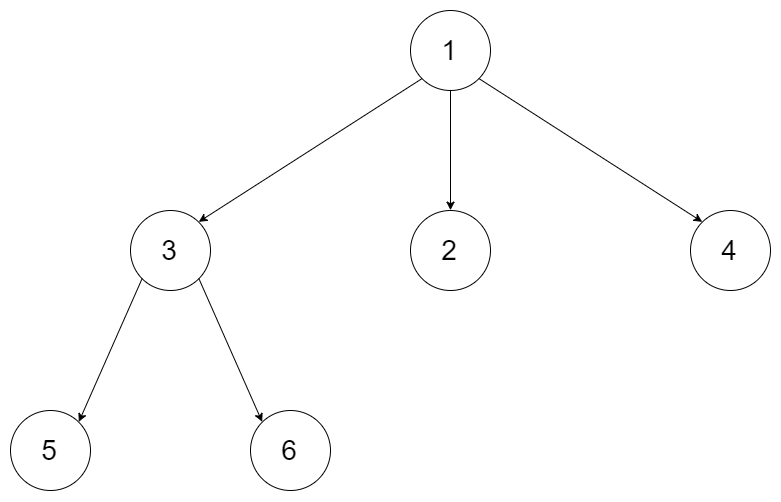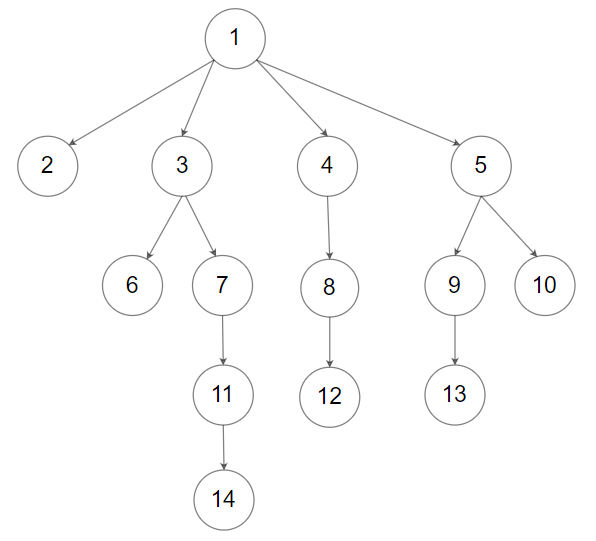# 559. Maximum Depth of N-ary Tree

Easy
Given a n-ary tree, find its maximum depth.
The maximum depth is the number of nodes along the longest path from the root node down to the farthest leaf node.
Nary-Tree input serialization is represented in their level order traversal, each group of children is separated by the null value (See examples).
Example 1:Input: root = [1,null,3,2,4,null,5,6]
Output: 3
Example 2:Input: root = [1,null,2,3,4,5,null,null,6,7,null,8,null,9,10,null,null,11,null,12,null,13,null,null,14]
Output: 5
Constraints:
• The total number of nodes is in the range `[0, 10^4]`.
• The depth of the n-ary tree is less than or equal to `1000`.

### 解題

/**
* Definition for a Node.
* type Node struct {
* Val int
* Children []*Node
* }
*/
func maxDepth(root *Node) int {
ans := 0
var dfs func(*Node, int)
dfs = func(r *Node, depth int) {
if r == nil { return }
if depth + 1 > ans { ans = depth + 1 }
for _, child := range r.Children {
dfs(child, depth + 1)
}
}
dfs(root, 0)
return ans
}